Next: Measurement of Ionospheric Electron Up: Wave Propagation in Inhomogeneous Previous: Extension to Oblique Incidence

# Ionospheric Pulse Propagation

Consider a radio wave generator that launches radio pulses vertically upwards into the ionosphere. For the sake of argument, we shall assume that these pulses are linearly polarized such that the electric field vector lies parallel to the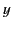-axis. The pulse structure can be represented as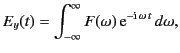(1117)

where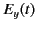is the electric field produced by the generator (i.e., the field at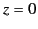). Suppose that the pulse is a signal of roughly constant (angular) frequency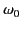that lasts a time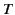, whereis long compared to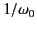. It follows that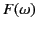possesses narrow maxima around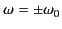. In other words, only those frequencies that lie very close to the central frequency,, play a significant role in the propagation of the pulse.

Each component frequency of the pulse yields a wave that travels independently up into the ionosphere, in a manner specified by the appropriate WKB solution [see Equations (1104)-(1105)]. Thus, if Equation (1119) specifies the signal at ground level () then the signal at height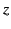is given by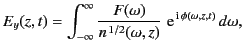(1118)

where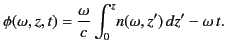(1119)

Here, we have made use of the definition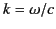.

Equation (1120) can be regarded as a contour integral in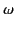-space. The quantity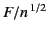is a relatively slowly varying function of, whereas the phase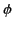is a large and rapidly varying function. As described in Section 7.12, the rapid oscillations of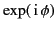over most of the path of integration ensure that the integrand averages almost to zero. However, this cancellation argument does not apply to those points on the integration path where the phase is stationary: that is, where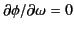. It follows that the left-hand side of Equation (1120) averages to a very small value, expect for those special values ofand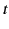at which one of the points of stationary phase in-space coincides with one of the peaks of. The locus of these special values ofandcan be regarded as the equation of motion of the pulse as it propagates through the ionosphere. Thus, the equation of motion is specified by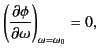(1120)

which yields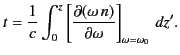(1121)

Suppose that the-velocity of a pulse of central frequencyat heightis given by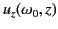. The differential equation of motion of the pulse is then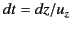. This can be integrated, using the boundary conditionat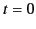, to give the full equation of motion: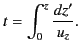(1122)

A comparison between Equations (1123) and (1124) yields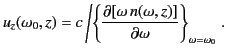(1123)

The velocity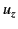corresponds to the group velocity of the pulse. (See Section 7.13.)

The dispersion relation (1056) yields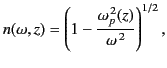(1124)

in the limit that electron collisions are negligible. The phase velocity of radio waves of frequencypropagating vertically through the ionosphere is given by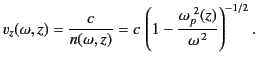(1125)

According to Equations (1125) and (1126), the corresponding group velocity is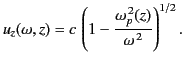(1126)

It follows that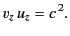(1127)

Note that as the reflection point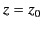[defined as the root of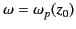] is approached from below, the phase velocity tends to infinity, whereas the group velocity tends to zero.

Let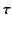be the time taken for the pulse to travel from the ground to the reflection level, and then back to the ground again. The product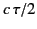is termed the equivalent height of reflection, and is denoted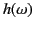, because it is a function of the pulse frequency,. The equivalent height is the height at which an equivalent pulse traveling at the velocity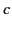would have to be reflected in order to have the same travel time as the actual pulse. Because we know that a pulse of dominant frequencypropagates at heightwith the-velocity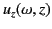(this is true for both upgoing and downgoing pulses), and also that the pulse is reflected at the height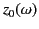, where, it follows that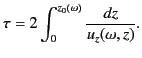(1128)

Hence,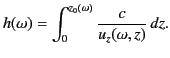(1129)

The equivalent height of reflection,, is always greater than the actual height of reflection,, because the group velocityis always less than the velocity of light. The previous equation can be combined with Equation (1128) to give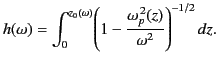(1130)

Note that, despite the fact that the integrand diverges as the reflection point is approached, the integral itself remains finite.Next: Measurement of Ionospheric Electron Up: Wave Propagation in Inhomogeneous Previous: Extension to Oblique Incidence
Richard Fitzpatrick 2014-06-27